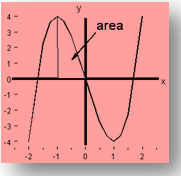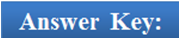×#### Thank you for registering.

One of our academic counsellors will contact you within 1 working day.

Click to Chat

1800-1023-196

+91-120-4616500

CART 0

• 0

MY CART (5)

Use Coupon: CART20 and get 20% off on all online Study Material

ITEM
DETAILS
MRP
DISCOUNT
FINAL PRICE
Total Price: Rs.

There are no items in this cart.
Continue ShoppingProperties of Definite IntegrationDefinite integration is an important component of integral calculus which generally fetches a good number of questions in various competitive exams. Both indefinite and definite integration are interrelated and indefinite integration lays the groundwork for definite integral. Students are advised to learn all the important formulae as they aid in answering the questions easily and accurately. We shall discuss here some of the important properties of definite integrals and then throw some light on their applications as well:Important Properties of Definite Integrals

Some of the basic yet important properties which prove fruitful while attempting questions on definite integral are listed below. These results can be proved easily, but the derivations are not very important. Students must remember all the results as the questions can’t be solved unless you know these results.

1.

2.

3.

4. Change of variable of integration is immaterial as long as the limits of integration remain the same, i.e.

5. If the limits are interchanged, i.e. the upper limit becomes the lower limit and vice versa, then

6. If f is a piecewise continuous function, then the integral is broken at the points of discontinuity or at the points where the definition of f changes, i.e.

7.

8.

Another result that can be derived from this property is

9.

10.

where f(T + x) = f(x), m ∈ I.

11.

12.

where f(x) is periodic with period ‘T’ and n ∈ I.

13.

where f(x) is periodic with period a.

14.

15. If f(x) ≥ 0 on the interval [a, b], then

16. Let f(x) and g(x) be two functions defined and continuous on [a, b], then1. Indefinite integral lays the foundation for definite integral. One cannot expect to excel in definite integration without mastering the concepts of indefinite integration.

2.

the equation f(x) = 0 has at least one root lying in (a, b) provided f is a continuous function in (a, b).

3. If f(x) ≤ g(x) for x ∈ [a, b] then

4. If f(x) ≥ 0 on the interval [a, b], then

We now have a look at some of the examples which illustrate the application of these properties:

Illustration 1:

Evaluate

Solution:

Value of tan x at x = 5π/12 is (2 + √3)

Value of tan x at x = 0 is 0

Integers between 0 and 2 + √3 are 1, 2, 3

∴ tan x = 1, tan x = 2, tan x = 3

⇒ x = tan-1 1, x = tan-1 2, x = tan-1 3

Hence, using these as the limits of integration and solving I we get,

=  0 + (tan–1 2 – tan–1 1) + 2 (tan–1 3 – tan–1 2) + 3 (5π /12 – tan–1 3)

=   5π/4 – π/4 – tan–1 3 – tan–1 2

=  π - [tan–1 {(3 + 2)/(1 – 6)}

= –tan-1 (-1)

= π/4

______________________________________________________________________

Illustration 2:

If f(x) is a function satisfying f(x + a) + f(x) = 0 for all x ∈ R and constant a such that ∫c+bb f(x) dx is independent of b, then find the least positive value of c.

Solution:

We have f(x + a) + f(x) for all x ∈ R         ……(i)

⇒ f(x + a + a) + f(x + a) = 0 [Replacing x by x + a]      ….(ii)

⇒ f(x + 2a) + f(x+ a) = 0      ….(iii)

Subtracting (i) from (iii), we get f(x + 2a) - f(x) = 0 for all x ∈ R.

⇒ f(x + 2a) = f(x) for all x ∈ R

So, f(x) is periodic with period 2a.

It is given that ∫c+bb f(x)dx is independent of b.

∴ The minimum value of ‘c’ is equal to the period of f(x) i.e., 2a.

_______________________________________________________________________________

Illustration 3:

What is the area under the curve y(x) = 2x3 - 6x between x = -1 and x = 0?Solution:

The required area A is given by

Solving this gives

0 – (1/2 – 3) = 5/2.

_____________________________________________________________________

Illustration 4:

Find the value of

Solution:

Let I = I1 + I2

….......... (1)

Solving this I1 can also be written as

…..... (2)

Adding (1) and (2) , we get

2I1 = ∫π0 dx / 1+ 5cosx + ∫π0 5cosx dx/(5cosx + 1) = π

I1 = π/2

Now,

I2 = ∫2–2 log (5–x / 5+x) dx

Let g(x) = log (5–x / 5+x)

Now g(-x) = log(5 – x / 5 + x) = –log (5–x/5+x) = –g(x)

∴ g(x) is an odd function

∴∫2–2 g(x)dx = 0 ⇒ I2 = 0

I = I1 + I2 =  π/2 + 0 = π/2

________________________________________________________________________Q1. Suppose the upper and the lower limits of integration are same, then the value of integral is

(a) 0

(b) 1

(c) can’t say

(d) positive

Q2. If the value of an integral is zero, then

(a) the function is zero.

(b) the function f has a root in (a, b)

(c) The function f is discontinuous in (a, b).

(d) if f is continuous in (a, b), then the equation f(x) = 0 has at least one root in (a, b).

Q3.

(a) always holds good.

(b) true only if f(x) is periodic with period ‘a’.

(c) can never be true.

(d) true for any integer ‘a’.

Q4. If f(x) ≥ 0, then

(a)

(b)

(c)

(d)

Q5.

(a) provided f(x) is periodic with period ‘T’ and n ∈ I.

(b) true for any n and T.

(c) this inequality cannot hold good in any case.

(d) can’t say.Q1(a), Q2(d), Q3(b), Q4(c), Q5(a).

Related Resources

For getting an idea of the type of questions asked, refer the previous year papers.

You may wish to refer indefinite integral.

To read more, Buy study materials of Definite integral comprising study notes, revision notes, video lectures, previous year solved questions etc. Also browse for more study materials on Mathematics here.### Course Features

• 731 Video Lectures
• Revision Notes
• Previous Year Papers
• Mind Map
• Study Planner
• NCERT Solutions
• Discussion Forum
• Test paper with Video Solution